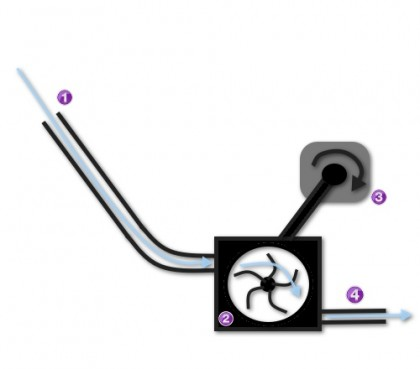# Hydroelectric Energy – How Hydroelectricity Works

Hydroelectric energy is produced when the kinetic energy of water is converted into electricity using a hydro turbine generator.

There are several methods for using water to power a hydro turbine generator, but they each generally function in a relatively similar manner, all using the same fundamental laws of physics.

And although there are numerous variables that can influence the design of each system, for example head height, water pressure, flow rate, and turbine design, the fundamental rule remains: that the hydro turbine generator is the device that converts the mechanical energy into electrical energy.

Once you understand the basics of how hydroelectricity works, that knowledge can be translated to the many varieties of hydroelectric energy systems.

## Example of how hydroelectricity works:Again, this is an example of a hydroelectric turbine works.  The device above illustrates a system with a high head height, as the water begins at point (1) and falls a long distance towards the center of the system.  These high head systems are usually found in geographical locations with frequent elevation changes, like a hilly or mountainous area, and requires less volume of water because the high vertical drop of the water supplies sufficient force to spin the turbine.

Let’s take a step-by-step look at what is happening in this hydroelectric system to create electricity.

1. The intake valve, sometimes called the penstock, is the part of the device that captures the flow of water and directs it towards the water turbine.  The water source may vary depending on the system, for example a man-made reservoir, or a natural source like a river or lake.
2. Then, the water makes its way towards a series of curved blades, which catch the force of the water as it passes through, which then causes the turbine to rotate.  The force of gravity is what supplies the falling water with mechanical energy, and the turbine blades are what absorb the energy as the water collides into them.
3. The turbine is then attached to a turbine generator which also rotates because it is attached by a rotating shaft to the spinning water turbine.  As the generator rotates, an electric current is created and can eventually be converted into usable electricity.
4. As the water passes through the spinning turbine, it is forced to exit through the exit valve as new water continuously falls through the machine to support a continuous flow of water.

Again, this is an example of how a high head hydroelectric energy turbine works, and the components and general physics of each other type is very similar.  All hydroelectric energy systems work by having flowing water move through a turbine blade system that is attached to a turbine generator.

Calculating Hydro-Power Output

Hydroelectric energy production accounts for almost one-quarter of the electricity used in the world, which is enough to supply around 1 billion people with electric power.

Hydroelectricity is the leading renewable energy resource currently in the United States, accounting for around 6% of all electricity produced, or about 70% of all electricity produced from sources of renewable energy.

The Hoover Dam, a well-known and very large hydroelectric dam in the United States, supports 17 main turbine generators and could produce over 2000 megawatts of electricity.

The Hoover Dam has generated, on average, around 4 billion kilowatt-hours of electricity per year which is enough energy to power almost 1.5 million people.

Small hydroelectric turbine generators usually produce up to 10 megawatts of power.  Micro hydroelectricity turbines will usually produce 100 kilowatts of electric power.

Two very important factors needed to calculate potential hydro power output of a hydroelectricity system are water flow and hydraulic head height.

Water flow is the volume of water that passes through the turbine in a specified amount of time, and head height is the distance that the water falls before reaching the turbine.

The greater the flow, or the larger the head height, the higher the potential output of hydroelectricity will be.

Gravity is the other component needed to complete the power output equation, but gravity is a constant (9.8), so it does not change.  With gravity and the two variables to equation to calculate hydroelectric power output is:

Power = Gravity*Water Flow Rate*Head Height

To receive the power output in watts, gravity is measure in meters per second squared, water flow is measured in liters per second, and head height is measured in meters.

It is now important to understand that the answer you get will be the power output in watts of a machine that converts 100% of the mechanical energy of water into hydroelectricity.  This, unfortunately, is never the case.  Hydroelectricity production usually works in a 50%-70% efficiency range.  So, by multiplying your answer by the efficiency rate of the machine, you will get the real calculated power output of your hydroelectric machine.